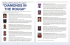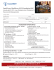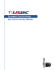Center of Gravity placement on a symmetrical bowling ball: How

Transcription

Center of Gravity placement on a symmetrical bowling ball: How
Center of Gravity placement on a symmetrical
bowling ball: How critical is it?
By Paul Ridenour, USBC research engineer
A hot topic on Internet forums recently has been the effect
of center of gravity placement in symmetrical bowling
balls. The two main pieces of evidence for this study are
videos
by
Brunswick
regional
Professional
Bowlers
Association staffer Nick Smith, whose video can be found at
brunsnick.com and Lane #1 president Richie Sposato, whose
video is at lane1bowling.com.
Those videos are effective visual evidence. For a more in
depth look, the USBC research staff decided to use robotic
ball thrower Harry to roll the balls.
gravity effect on ball motion and will give preliminary
conclusions observed from C.A.T.S. data.
When bowling ball companies design bowling balls in CAD
programs, they usually have a certain pin to center of
gravity distance in mind. Because of variation in the
manufacturer’s process, USBC receives pin out distances
that vary from 1 inch to sometimes 6 inches. When bowling
balls are marketed, the figures for total differential and
radius of gyration are completed from the model of that
particular ball on a CAD program. According the some ball
manufacturers, the statistical numbers of a bowling ball
can vary from the CAD numbers by about plus or minus 3
percent. The only way to truly measure those numbers once a
ball is made is to use a radius of gyration swing such as
the one USBC has to test bowling balls.
Once a bowling ball is drilled, those measurements taken
before the ball was drilled will change, by also about plus
or minus 5 percent depending on the drilling technique. If
a weight hole was added to the ball to account for static
weight,
the
total
differential
and
intermediate
differential of that ball will change depending on the
size, pitch, amount of core material removed and placement
of the hole. An un-drilled symmetrical bowling ball only
has one low radius of gyration point, and it has a high
radius of gyration equator. That equator is present because
the radius of gyration of both y and z axes are equal to
one another. This does not apply to asymmetrical balls, for
which the high radius of gyration spot is marked as the
“mass bias” 95 percent of the time.
three axes, USBC research engineers also measured the
which is 5 inches over by 3/8 inch up. USBC used two
Columbia 300 Wrath SF balls with 3 ounces of top weight and
2-2.5 inch pin to CG distances for the two test balls. The
pin for both of these balls was located in the midline of
the grip. The high RG equator was located through the thumb
before drilling and after drilling both balls spun the
exact same spot. The only difference in the two balls was
the center of gravity placement which was done at a 45degree angle away from the midline. Photos of the balls are
shown below.
Figure 1: Photos of the positive CG ball and negative CG
balls used in the test.
The critical element of the experiment is the exact
location for the pin because that will determine the
axis point. After properly drilling the two bowling balls
shown in Figure 1, the numbers for the two balls are fairly
similar. Measurements are shown below for both the positive
and negative in Figure 2.
Positive CG ball
2.518
Negative CG ball
2.514
of
Gyration for x
axis
Total
0.047
0.045
Differential
Intermediate
0.009
0.008
Differential
of 2.554
2.548
Gyration
on
the PAP
Figure 2: Post-drilling data from the two bowling balls.
The data from the graphs in Figure 2 are very close to one
another. USBC experience has determined that the naked eye
cannot distinguish the ball’s reaction from these balls
alone. In addition to these figures, the top weights are
relatively the same; the only substantial difference is
that the positive CG ball has positive side weight of 1.25
ounces and the negative CG ball has negative side weight of
1.35 ounces. The tests were all performed using Harry,
rolling the balls at 17 mph and 375 rpm. The revolution
rate was increased from the normal ball test to enhance the
differences one would see in the reaction of these balls.
The standard 53-foot oil pattern used for the USBC Ball
Motion Study was applied for an eight-shot test. That
pattern is flat with about 30 units of oil at eight feet,
12 units at 32 feet and four to six units at 51 feet. This
type of alternating “ABBABAAB” test allows each ball a
theoretically equal amount of oil time on the lanes. One
eight-shot test was used as a break-in period for the two
balls in the study.
30
Ball A Equations
y = -0.3071x + 14.993
R2 = 0.9938
y = 0.0165x2 - 0.988x + 22.095
R2 = 0.9876
'11'-23' Ball A
y = 0.7819x - 23.702
R2 = 0.99
23'-41' Ball A
25
Position from the Right Gutter (in)
41'-60' Ball A
11'-25' BallB
20
25'-47' Ball B
47'-60' Ball B
15
Linear ('11'-23' Ball A)
Poly. (23'-41' Ball A)
10
Linear (41'-60' Ball A)
Linear (11'-25' BallB)
5
Ball B Equations
y = -0.3048x + 15.037
R2 = 0.9901
Poly. (25'-47' Ball B)
y = 0.0155x2 - 0.9667x + 22.165
R2 = 0.9988
y = 0.8672x - 30.228
R2 = 0.994
Linear (47'-60' Ball B)
0
0
10
20
30
40
50
60
70
Position from the Foulline (ft)
Figure 3: Ball motion data on the positive CG vs. negative
CG ball.
Figure 3 illustrates the position graph from the C.A.T.S.
data obtained during the eight-shot test. Each path
represents an average of the four shots for that particular
ball. Ball A is the positive center of gravity ball, ball B
is the negative center of gravity ball. It is worth noting
that although the two graphs line up at the beginning with
each other, the negative center of gravity ball takes four
feet longer to start its hook phase compared to the
positive center of gravity ball.
From the ball motion study, USBC studies 20 different
variables in terms of ball motion, shown in the chart
below. Notable on the chart is that the positive center of
gravity ball was the maximum in 14 categories compared to
four for the negative center of gravity ball with two ties.
Some key statistics from this test are that the positive
center of gravity ball is two boards stronger on the back
end in the oil than the negative center of gravity ball and
the positive CG ball is a foot and a quarter sooner than
the negative CG ball. For a definition of these 20 Ball
Motion Study variables, please see the Ball Motion Study
Power Point presentation posted on bowl.com.
Ball motion statistics
Average Difference in Intended
Path @ 49’
Average Difference in Intended
Path @ 60’
Difference in Average Path @ 49’
Difference in Average Path @ 60’
Velocity Decrease from 13’-49’
Velocity Decrease from 13’-60’
Change in Angle To Headpin @
13’-49’
Change in Angle To Headpin @
13’-60’
Angle Statistics
Position of 1st Transition (ft)
Position of 2nd Transition (ft)
Skid Phase Slope
Hook Phase Slope
Total Angle Displacement
Total Hook Phase Length
Change in Angle/Foot in Hook
Phase
A Value in Polynomial Section
Breakpoint of Polynomial Section
Length from 1st Transition to
Breakpoint
Length from 2nd Transition to
Breakpoint
Frictional Efficiency
Wrath
Pos
Wrath
Neg
“Category
Max”
17.27
15.18
Wrath Pos
29.90
17.19
30.31
1.90
2.69
28.20
15.33
28.62
1.67
2.53
Wrath
Wrath
Wrath
Wrath
Wrath
6.06
5.81
Wrath Pos
7.18
7.13
Tie
23
41
-0.307
0.782
55.09
18
25
47
-0.305
0.867
57.88
22
Wrath
Wrath
Tie
Wrath
Wrath
Wrath
3.061
2.631
Wrath Pos
0.0165
29.94
0.0155
31.18
Wrath Pos
Wrath Pos
6.94
6.18
Wrath Neg
11.06
0.0538
15.82
0.0476
Wrath Pos
Wrath Pos
Pos
Pos
Pos
Pos
Pos
Pos
Pos
Neg
Neg
Neg
Figure 4: Ball Motion Study Statistics
It is worth noting that even though these are minor
differences, they are still differences. Mathematically,
the difference in position is roughly only about 10
percent; this is not always easy to tell on the lanes
observing from 60 feet away. USBC had thought the balls
looked very similar in reaction; however, the math paints a
different picture. Also realize that this is only one test,
and that additional tests must be conducted to verify that
these results are consistent for most bowling balls. USBC
has performed some calculations to show that the static
measurements of a drilled ball may affect between 3 to 8
percent of overall ball motion.
In Phase 2 of the USBC Ball Motion Study, static weight
measurements are being added as an x, or static, variable.
Since all balls are drilled the same in the ball motion
study, static weights may not stand out. That is why USBC
has also prioritized an in depth static weight test to be
completed after the study. This test should start in early
2008. The Ball Motion Study’s statistical analysis will
investigate static weight’s overall percentage of influence
over total ball motion. However, USBC’s concern is that the
Ball Motion Study’s static weights will be too similar to
see a real difference, hence another reason for the 2008
testing.
In closing, both sides of the center of gravity debate
should be able to appreciate this study, since it does show
a difference between positive weight and side weight;
however, that difference only amounts to about a 10 percent
difference overall in change of position on the lanes.

Carousel Carnival Poster 2015 - Petaluma Valley Athletic Club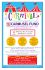Digital Patterns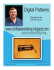FIVB Technical Posters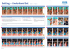Guru Suggested Asymmetrical Layouts copy (5)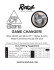Great Ideas_2011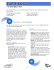Ball Exercise Chart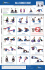STEM and Project-Based Learning: What`s All the Excitement About?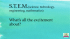diamonds in the rough - International Basketball League